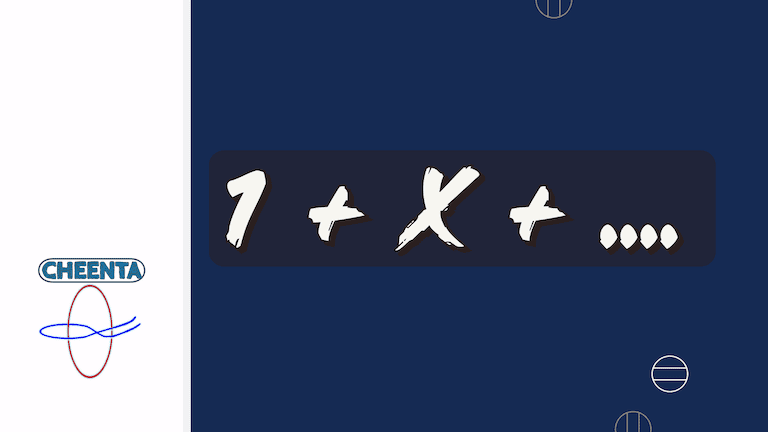How Cheenta works to ensure student success?
Explore the Back-Story

# Primes and Polynomials from I.S.I. EntranceTry the problem

This problem of primes and polynomials is from B.Stat, B.Math Entrance.

Let x and n be positive integers such thatis a prime number. Then show that n is a prime number.

Watch the video

Try the problem

This problem of primes and polynomials is from B.Stat, B.Math Entrance.

Let x and n be positive integers such thatis a prime number. Then show that n is a prime number.

Watch the video

This site uses Akismet to reduce spam. Learn how your comment data is processed.

### Knowledge Partner